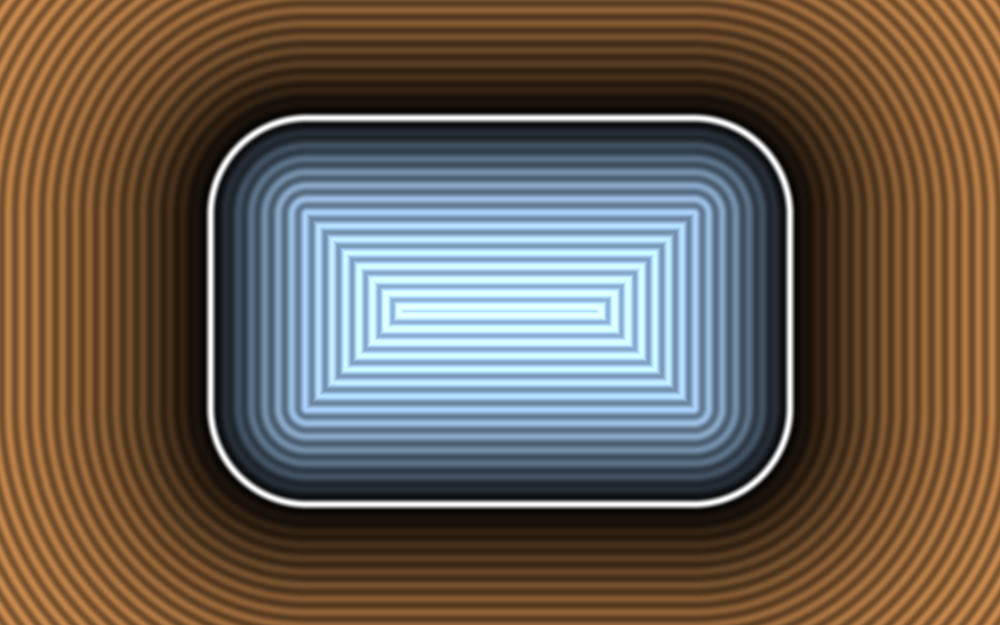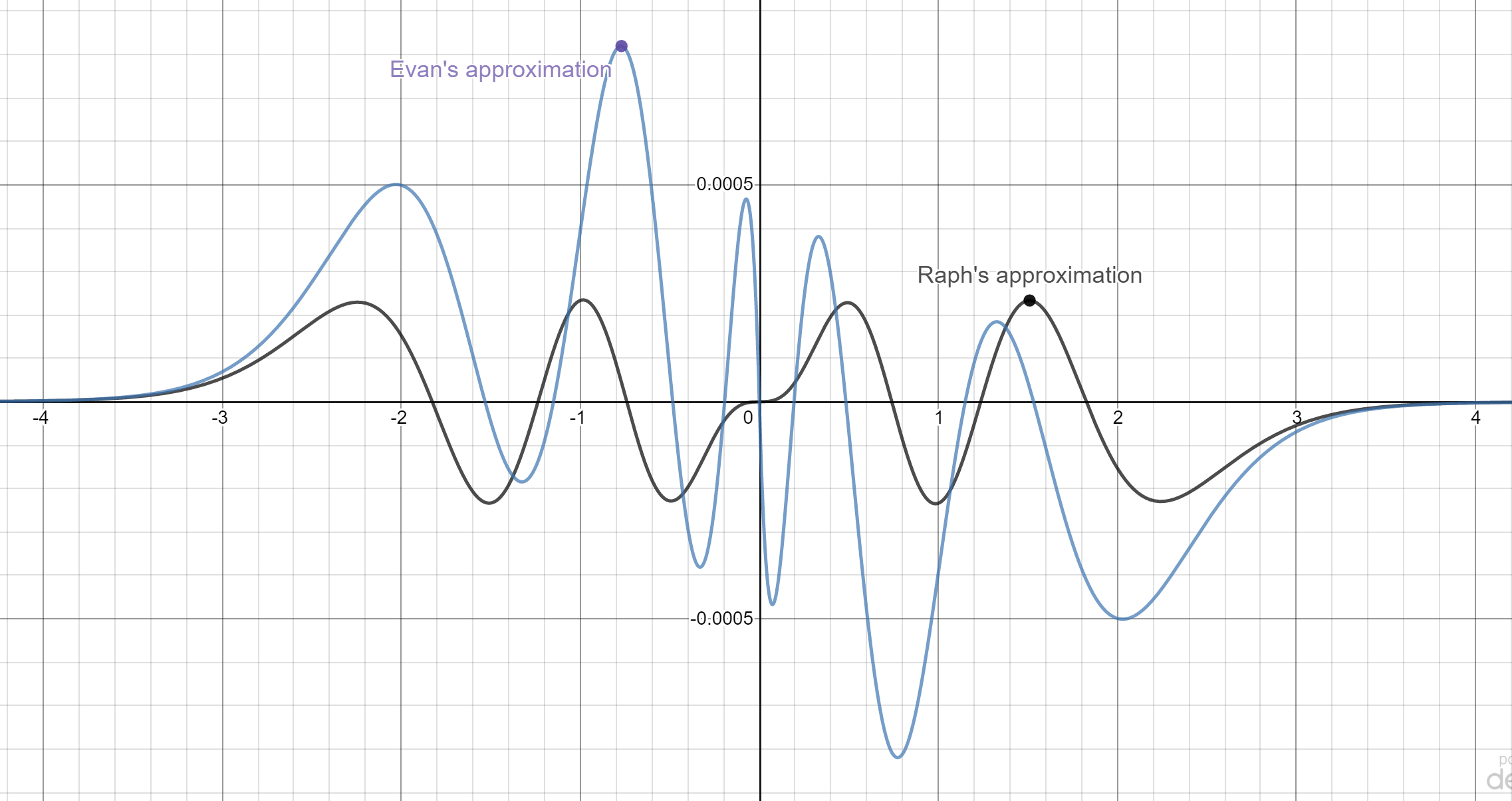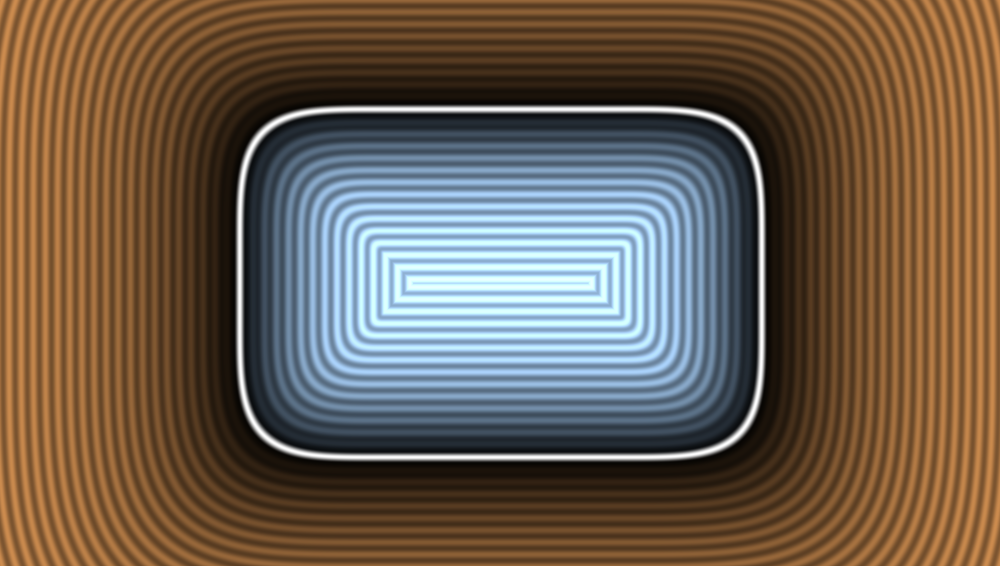Note: I’m publishing this with inadequate visuals, as it’s been stuck in my queue for 3 weeks and I want to get it out there. I’d like to return to making proper images, but make no promises when.

For now, a quick comparison of exact (computed with numerical integration) results (on the left) with my approximation:There are two basic ways to render blur in 2D graphics. The general technique is to render the objects into an offscreen buffer, compute a blur, and composite that into the target surface. But in special cases, it’s possible to compute the blurred image directly from the source object, which is much faster.

Some shapes are easy, particularly rectangles; they have a straightforward closed-form analytical solution. But others require numerical approximations. A few years ago, Evan Wallace posted a solution for fast rounded rectangle shadows, using an analytical solution in one direction and numerical integration in the other. This is a good solution, but I was curious whether it is possible to do better.

The solution in this blog post is based on distance fields, a very powerful technique that has been getting more attention because of it adapts so well to GPU evaluation in shaders. Inigo Quilez has been making elaborate 3d scenes built up out mostly out of distance field primitives, a stunning demonstration of the power and flexibility of the technique. This post will sketch out the development of a less artistic but still hopefully useful application. I enjoy playing with the underlying math, and hope this blog post will be educational or at least entertaining for some of my readers.

Developing this required exploring a lot of possibilities, as well as navigating through parameter spaces. It’s most common to use Jupyter notebooks, a JavaScript-based platform such as observable, or a comparable tool. But for this, partly to try it out, I tried Rust, building a simple visualizer application using druid, a cross-platform GUI toolkit. You can try the Web version, ported by Paul Miller.

## The 1D case: blurred box function

As a warmup, let’s take the one dimensional case, especially as we’ll be using it as the foundation of the 2D solution. The one dimensional analog of a rectangle is a boxcar function.

Gaussian blur is the convolution of a Gaussian bump with the underlying image. The convolution of a box with a Gaussian has a straightforward analytical solution. A boxcar is the difference of two step functions (offset by the thickness of the line), and the convolution of a step and a Gaussian is erf. Thus, the blurred image is the difference of two erf evaluations.

It happens that this solution generalizes to a rectangle. Since a rectangle is the outer product of two box functions, a blurred rectangle is the outer product of their blurs. However, we won’t be using this, as we’re concerned with rounded rectangles, which aren’t separable in this way.

## Distance field of a rounded rect

Instead, we’ll use distance functions, as they do have the power and flexibility we need.

The general approach is to compute a signed distance from an outline, then use that distance as input to a function which computes the actual grayscale value. This approach separates the problem into the shape of the contour lines and the values, which (for reasons we’ll see) are best understood as a cross-section through the minor axis of the rectangle.

As Jonathan Blow has recently tweeted, “The most useful thing I ever learned, about how to do geometric operations in software, is to separate the problem into parallel and orthogonal components. It applies to just about everything.” While this is most obvious for classical geometric problems such as projecting a point onto a line, distance field techniques can be seen as another tool in the toolbox following this general principle. A distance field represents the value of the orthogonal component, with the parallel component filtered out.

To visualize contours (the parallel component) better, we’ll quantize the grayscale values. And we can see that for relatively small blur radii these contours look a lot like plain rounded rectangles. This motivates the first solution:

• The curve is a rounded rectangle.

• The corner radius is computed as a combination of the original corner radius and blur radius.

• The cross-section of the minor axis is the 1D solution.

The combination cited in the second step is $\sqrt{r_c^2 + 1.25 r_b^2}$. The choice of this formula is motivated by the rule for the probability distribution of a sum of Gaussians, with the constant factor chosen empirically.

### Implementation

The distance field for a rounded rectangle can be computed exactly, and Inigo Quilez includes the formula in his catalog of 2D distance functions. In shader language:

float sdRoundedBox( in vec2 p, in vec2 b, in float r ) {
vec2 q = abs(p)-b+r;
return min(max(q.x,q.y),0.0) + length(max(q,0.0)) - r;
}(Image adapted from https://www.shadertoy.com/view/4llXD7)

Note the use of min and max rather than conditional branching. The former is much faster in both shaders and SIMD evaluation.

For erf, we’ll use an approximation. It’s one of my favorite sigmoids and we’ll use the techniques from that blog post.

pub fn compute_erf7(x: f64) -> f64 {
let x = x * std::f64::consts::FRAC_2_SQRT_PI;
let xx = x * x;
let x = x + (0.24295 + (0.03395 + 0.0104 * xx) * xx) * (x * xx);
x / (1.0 + x * x).sqrt()
}


Evan’s version is based on an approximation from Abramowitz and Stegun, which has similar accuracy and likely similar performance, but I like using reciprocal square root - it is particularly well supported in SIMD and GPU and is generally about the same speed as simple division.### Evaluation

And this does indeed work well for small blur radii, compared to the size of the rectangle and the corner radius. But as thr blur radius goes up, we start to see problems. For one, the corner radius gets smaller, achieving a sharp corner in the visible region. For two, the rounded parts butt against the smooth parts rather than joining smoothly.

## Squircles to the rescue

The contour of the blurred rounded rectangle strongly resembles a squircle or superellipse. Such a shape would solve both these problems.

Here what we want to do is adapt the distance field approach to use a distance-like metric rather than an exact distance to the reference curve. Basically, the game plan is as follows:

• Structure of distance field is same as rounded rect.

• Increase exponent from 2 (circle) to make superellipse shape.

• Cross-section is as above.

Increasing the exponent clearly solves the main issues with the pure rounded rectangle shape, namely the sharp interior corners (which generate a visible “x” structure) and the abrupt straight to curved transitions:A more complete writeup of the final code is a TODO for this blog (along with better visuals), but see the code for the detailed solution.

### Further refinements

As the blur radius goes up, two factors degrade the accuracy of the above solution. For one, the height of the peak in the real solution decreases faster than the 1D case. This is fixed with a constant scale multiplier, derived from the erf of the rectangle’s major axis. For two, the overall shape becomes less eccentric, more like a circle (in the limit, it becomes a radially symmetric blur function). This is fixed by subtracting a correction factor from the major (long) axis of the rectangle.

With these corrections in place, the approximation becomes quite accurate over the entire range of parameters. Accuracy is nearly perfect for the original use case - shadows for UI objects, but visually acceptable everywhere.

## Future work

A good solution to the blurred rounded rectangle problem is nice but perhaps not that exciting by itself; Evan’s existing solution is almost certainly good enough for most practical uses.

One obvious generalization is to more shapes. The easiest by far is to squircle-based rounded rectangle shapes, as these can almost certainly by accomplished by tuning the parameters on the existing pixel shading logic. A case can be made that squircles are better than classical rounded rectangles (certainly Apple thinks so). And the shader can readily be adapted to render both filled and stroked versions of the shape with high quality antialiasing.

Good approximations to many other blurred shapes are possible, as a rich set of 2D distance functions are known and in widespread use in shader circles.

Also, perhaps your designer prefers bokehlicious discs to Gaussian shadows. Doable. Just use a different cross-section function and tweak the parameters.

Some fine-tuning on the code can still be done. For example, the “magic constants” were mostly determined through experimentation. A more systematic approach would be to do a global optimization, minimizing the value of some error norm over a range of parameters. Maybe an enterprising reader will take this on!

## Thanks

Thanks to Evan Wallace for permission to use his WebGL code (hoped for in a future revision), to Jacob Rus for discussion about the math, and Paul Miller for the wasm port.